Documentation

### This is machine translation

Mouseover text to see original. Click the button below to return to the English version of the page.

## Poisson's Equation on Unit Disk

This example shows how to numerically solve a Poisson's equation, compare the numerical solution with the exact solution, and refine the mesh until the solutions are close.

The example uses the `solvepde` function. For the PDE Modeler app solution, see Poisson's Equation on Unit Disk: PDE Modeler App. Because the app and the programmatic workflow use different meshers, they yield slightly different results.

The Poisson equation on a unit disk with zero Dirichlet boundary condition can be written as $-\Delta u=1$ in $\Omega$, $u=0$ on $\delta \Omega$, where $\Omega$ is the unit disk. The exact solution is

`$u\left(x,y\right)=\frac{1-{x}^{2}-{y}^{2}}{4}.$`

For most PDEs, the exact solution is not known. However, the Poisson's equation on a unit disk has a known, exact solution that you can use to see how the error decreases as you refine the mesh.

### Problem Definition

Create the PDE model and include the geometry.

```model = createpde(); geometryFromEdges(model,@circleg);```

Plot the geometry and display the edge labels for use in the boundary condition definition.

```figure pdegplot(model,'EdgeLabels','on'); axis equal```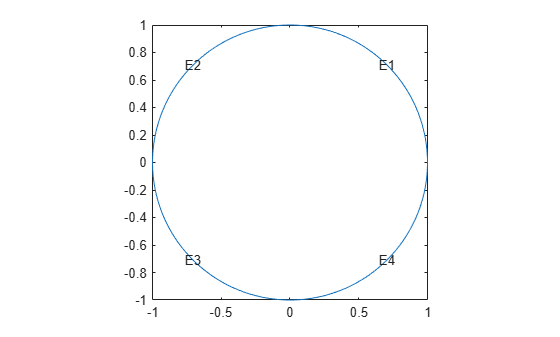Specify zero Dirichlet boundary conditions on all edges.

`applyBoundaryCondition(model,'dirichlet','Edge',1:model.Geometry.NumEdges,'u',0);`

Specify the coefficients.

`specifyCoefficients(model,'m',0,'d',0,'c',1,'a',0,'f',1);`

### Solution and Error with a Coarse Mesh

Create a mesh with target maximum element size 0.1.

```hmax = 0.1; generateMesh(model,'Hmax',hmax); figure pdemesh(model); axis equal```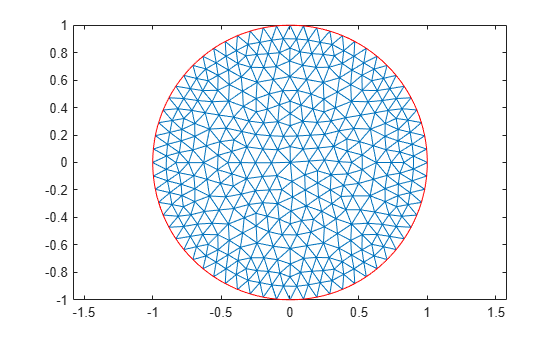Solve the PDE and plot the solution.

```results = solvepde(model); u = results.NodalSolution; pdeplot(model,'XYData',u) title('Numerical Solution'); xlabel('x') ylabel('y')```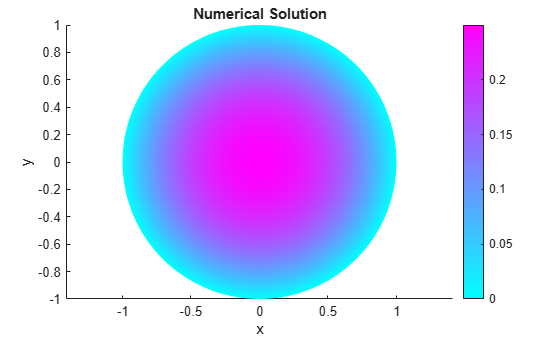Compare this result with the exact analytical solution and plot the error.

```p = model.Mesh.Nodes; exact = (1 - p(1,:).^2 - p(2,:).^2)/4; pdeplot(model,'XYData',u - exact') title('Error'); xlabel('x') ylabel('y')```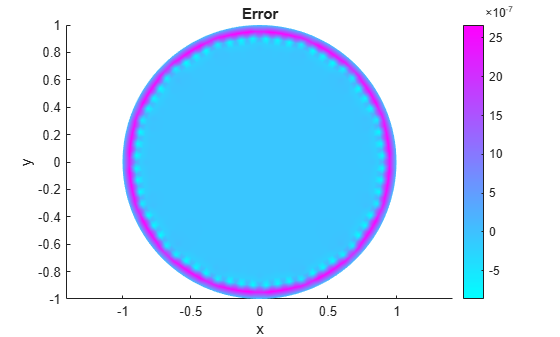### Solutions and Errors with Refined Meshes

Solve the equation while refining the mesh in each iteration and comparing the result with the exact solution. Each refinement halves the `Hmax` value. Refine the mesh until the infinity norm of the error vector is less than ${5\cdot 10}^{-7}$.

```hmax = 0.1; error = []; err = 1; while err > 5e-7 % run until error <= 5e-7 generateMesh(model,'Hmax',hmax); % refine mesh results = solvepde(model); u = results.NodalSolution; p = model.Mesh.Nodes; exact = (1 - p(1,:).^2 - p(2,:).^2)/4; err = norm(u - exact',inf); % compare with exact solution error = [error err]; % keep history of err hmax = hmax/2; end```

Plot the infinity norm of the error vector for each iteration. The value of the error decreases in each iteration.

```plot(error,'rx','MarkerSize',12); ax = gca; ax.XTick = 1:numel(error); title('Error History'); xlabel('Iteration'); ylabel('Norm of Error');```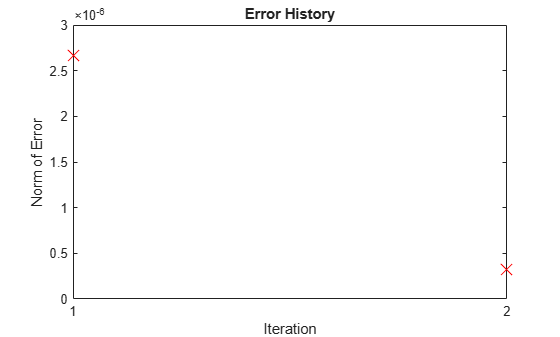Plot the final mesh and its corresponding solution.

```figure pdemesh(model); axis equal```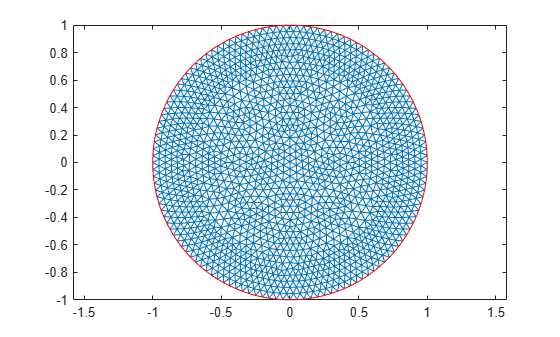```figure pdeplot(model,'XYData',u) title('Numerical Solution'); xlabel('x') ylabel('y')```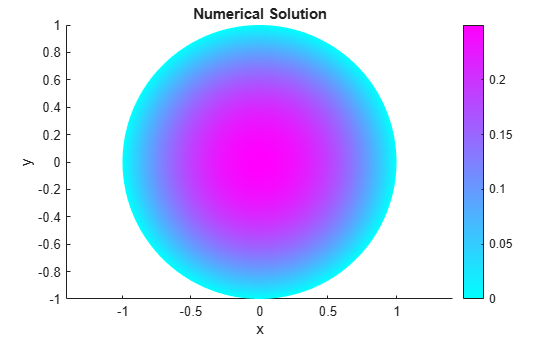Compare the result with the exact analytical solution and plot the error.

```p = model.Mesh.Nodes; exact = (1 - p(1,:).^2 - p(2,:).^2)/4; pdeplot(model,'XYData',u - exact') title('Error'); xlabel('x') ylabel('y')```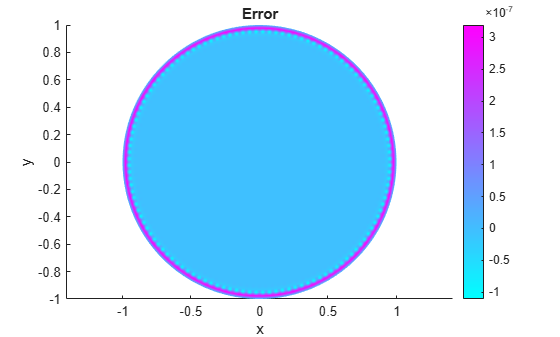##### SupportGet trial now